# NCERT Solutions for Class 10 Maths Exercise 7.2

Get Free NCERT Solutions for Class 10 Maths Chapter 7 Ex 7.2 Coordinate Geometry Class 10 Maths NCERT Solutions are extremely helpful while doing homework. Exercise 7.2 Class 10 Maths NCERT Solutions were prepared by Experienced ncertlive.in Teachers. Detailed answers of all the questions in Chapter 7 Maths Class 10 Coordinate Geometry Exercise 7.2 Provided in NCERT Textbook
NCERT Solutions for Class 10 Maths Chapter 7 Coordinate Geometry Ex 7.2 are part of NCERT Solutions for Class 10 Maths. Here we have given NCERT Solutions for Class 10 Maths Chapter 7 Coordinate Geometry Ex 7.2.

## NCERT Solutions for Class 10 Maths Exercise 7.2

Exercise 7.2 Class 10 Question 1
Find the coordinates of the point which divides the join of (- 1, 7) and (4, – 3) in the ratio 2:3.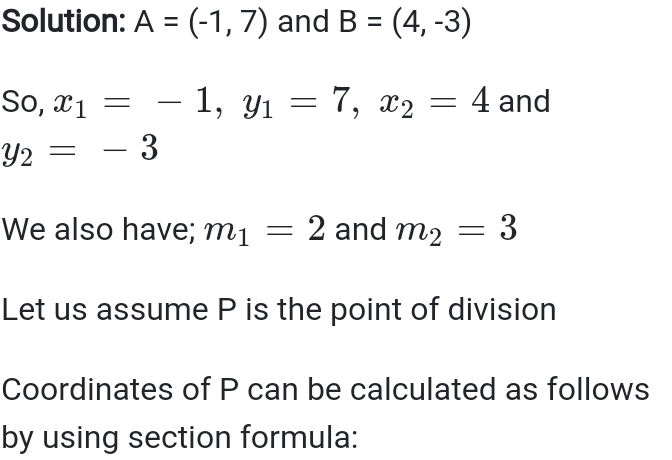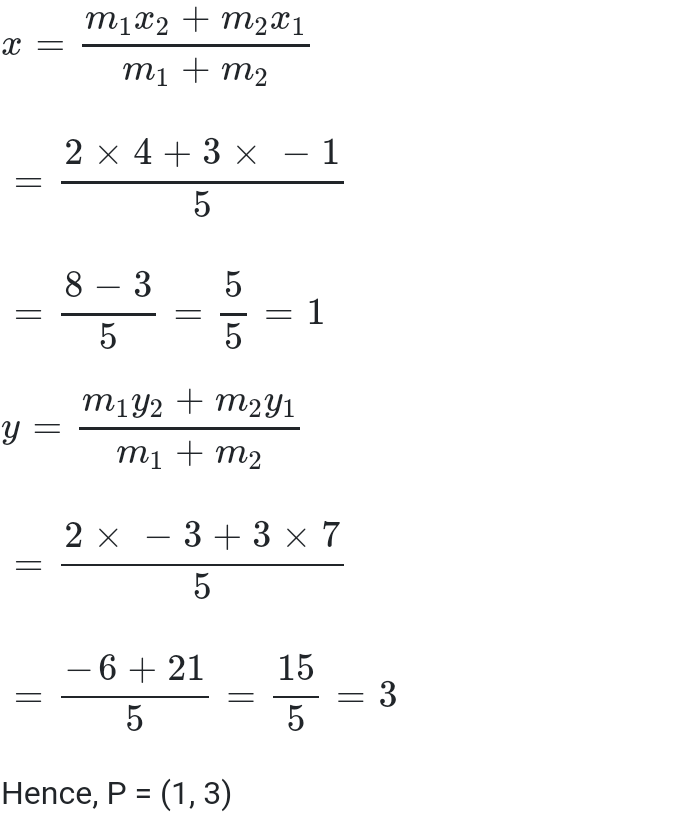Exercise 7.2 Class 10 Question 2
Find the coordinates of the points of trisection of the line segment joining (4, -1) and (-2, -3).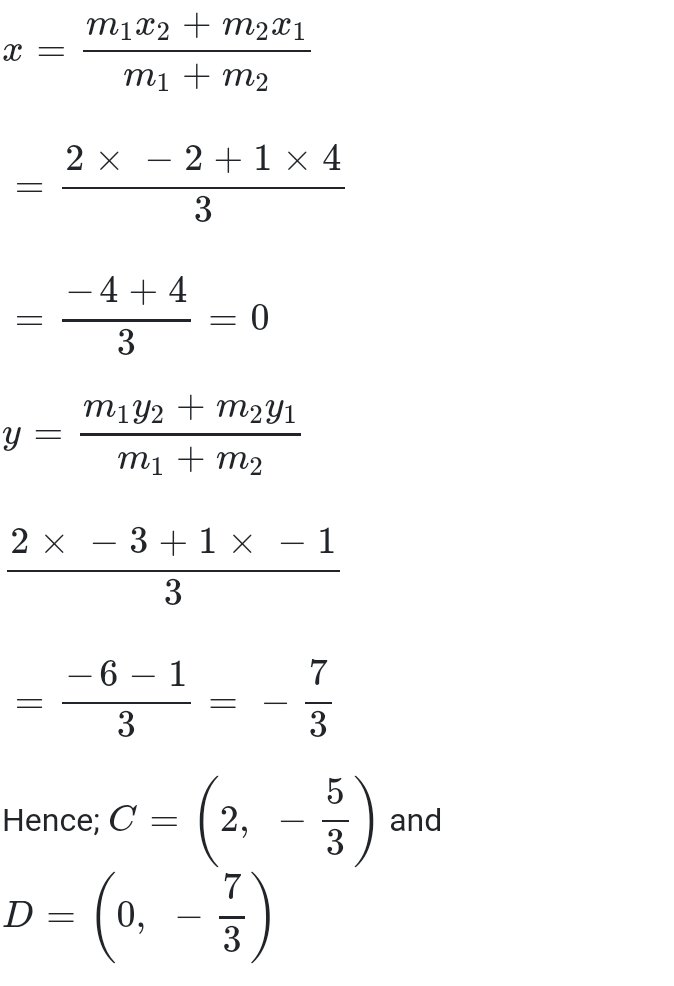Exercise 7.2 Class 10 Question 3
To conduct Sports Day activities, in your rectangular shaped school ground ABCD, lines have been drawn with chalk powder at a distance of 1 m each. 100 flower pots have been placed at a distance of 1 m from each other along AD, as shown in the following figure. Niharika runs 1/4 th the distance AD on the 2nd line and posts a green flag. Preet runs 1/5th the distance AD on the eighth line and posts a red flag. What is the distance between both the flags? If Rashmi has to post a blue flag exactly halfway between the line segment joining the two flags, where should she post her flag?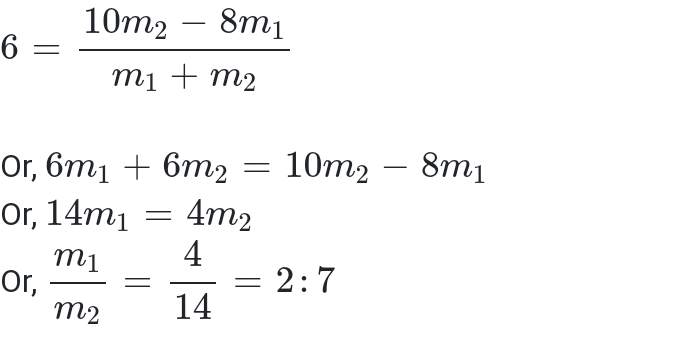Exercise 7.2 Class 10 Question 5
Find the ratio in which the line segment joining A (1, – 5) and B (- 4, 5) is divided by the x-axis. Also find the coordinates of the point of division.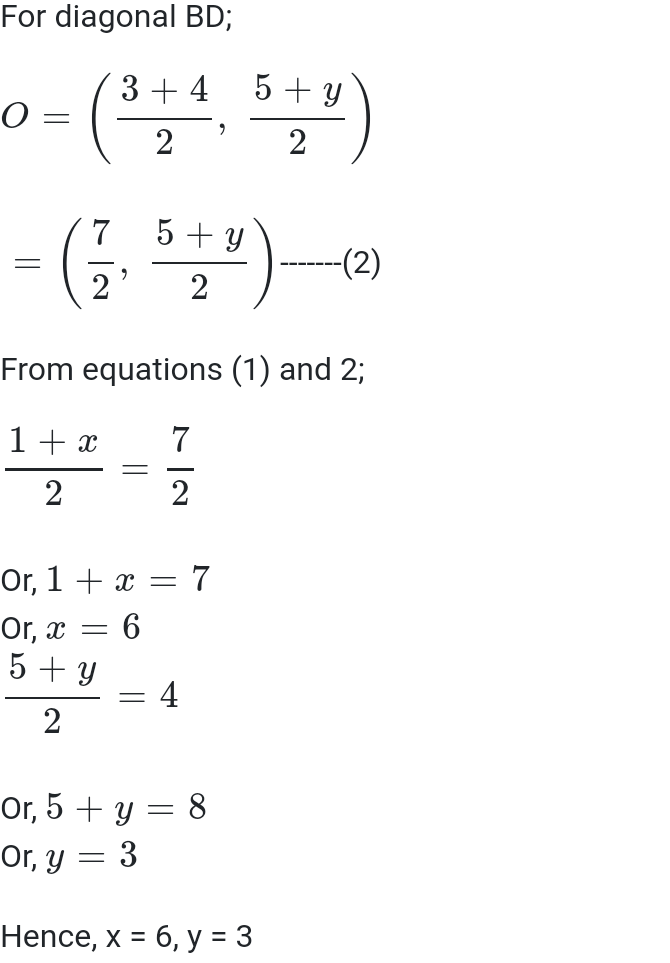Exercise 7.2 Class 10 Question 7
Find the coordinates of a point A, where AB is the diameter of circle whose centre is (2, – 3) and B is (1, 4).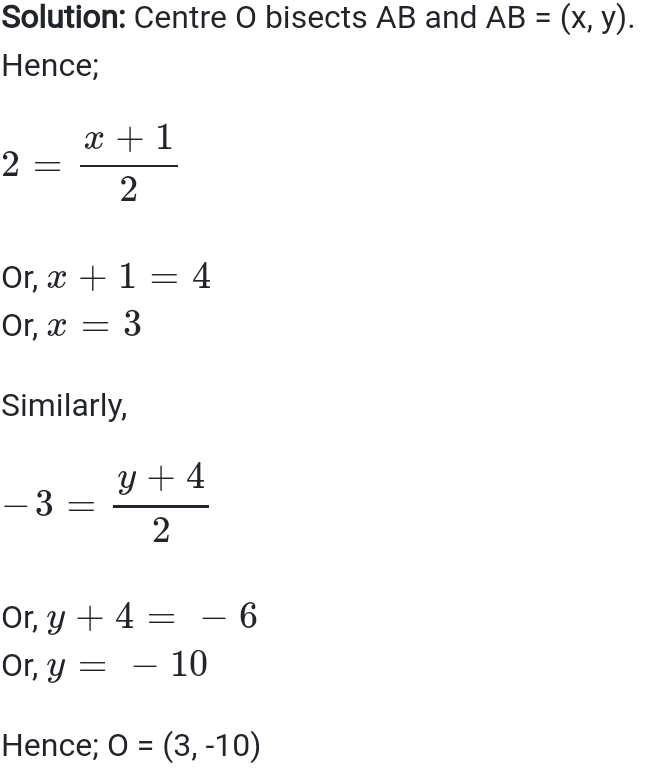Exercise 7.2 Class 10 Question 8
If A and B are (-2, -2) and (2, -4), respectively, find the coordinates of P such that AP = 3/7 AB and P lies on the line segment AB.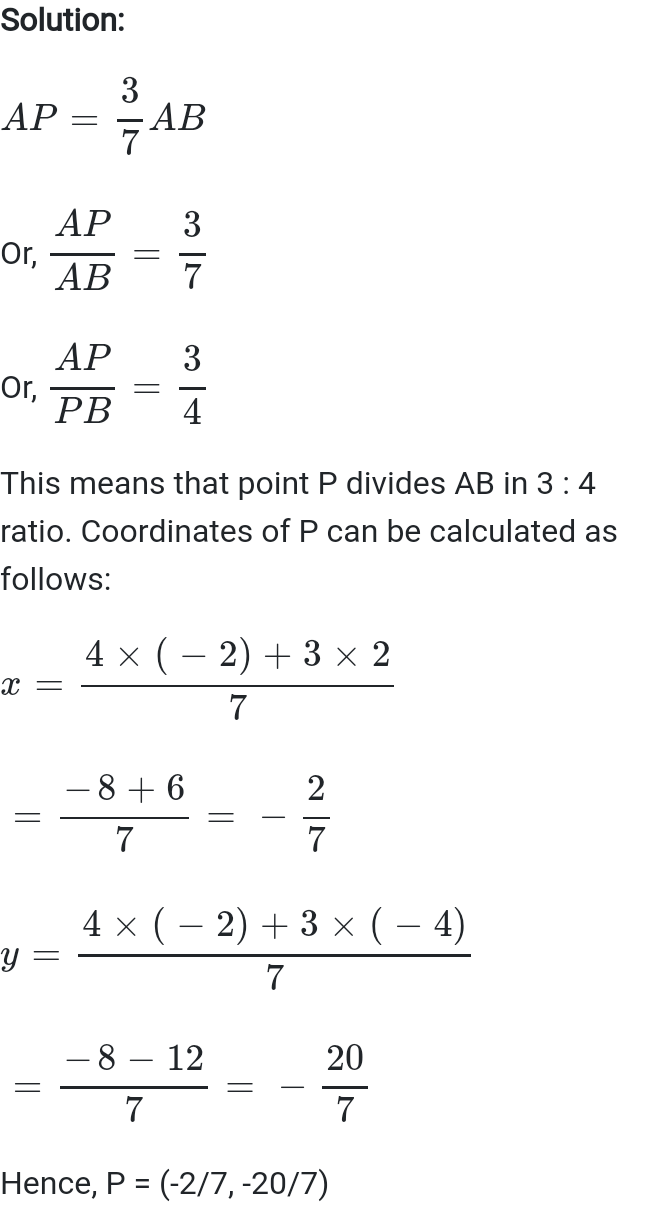Exercise 7.2 Class 10 Question 9
Find the coordinates of the points which divide the line segment joining A (- 2, 2) and B (2, 8) into four equal parts.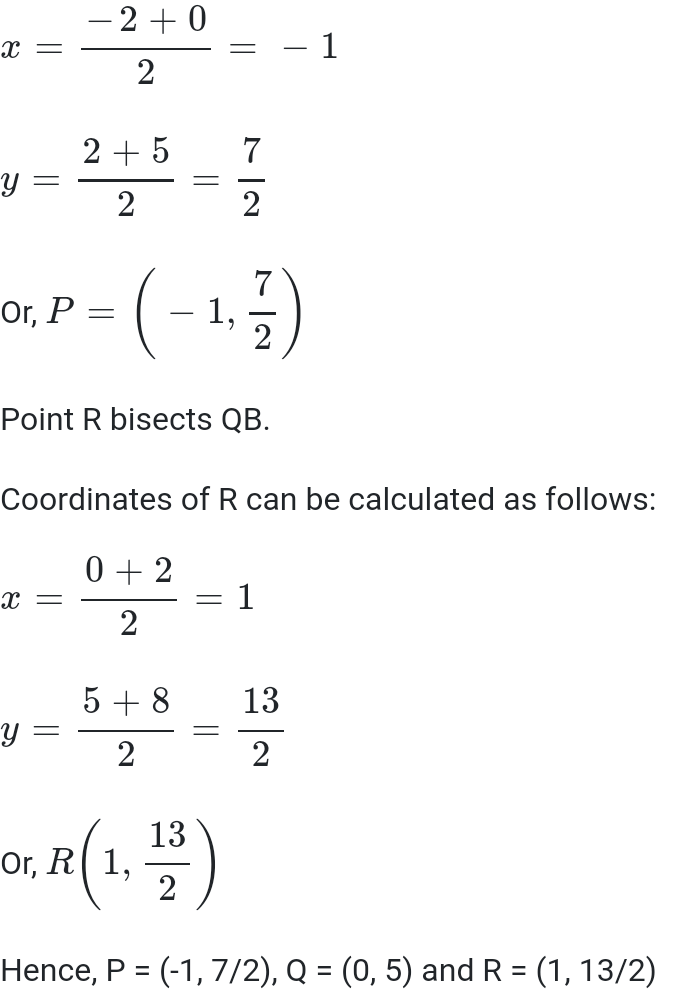Exercise 7.2 Class 10 Question 10
Find the area of a rhombus if its vertices are (3, 0), (4, 5), (-1, 4) and (-2,-1) taken in order. [Hint: Area of a rhombus = 1/2 (product of its diagonals)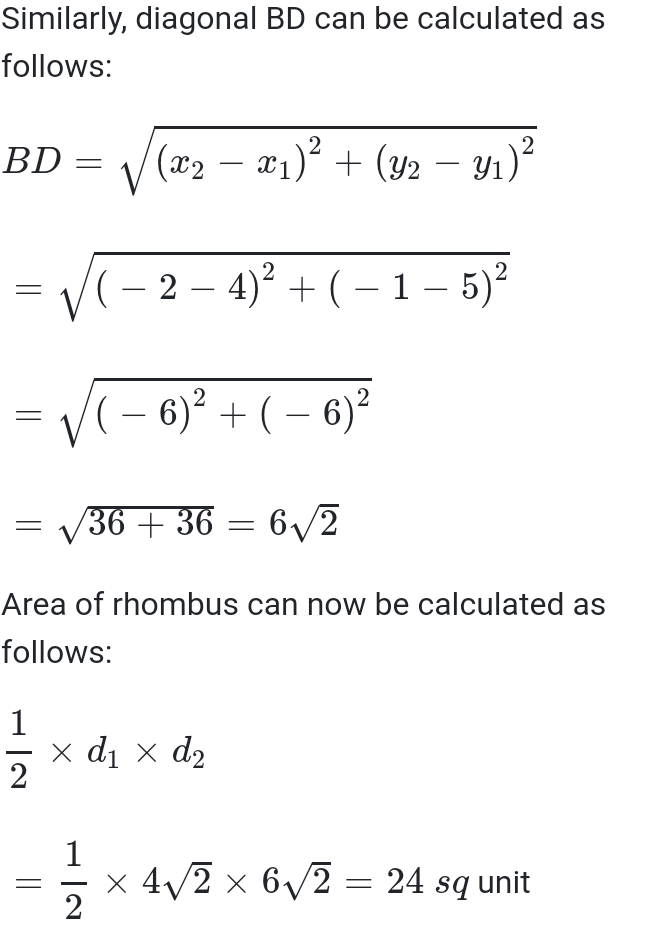Access other exercise solutions of Class 10 Maths Chapter 7-Coordinate Geometry
Exercise 7.1– 10 Questions (8 practical based questions, 2 reasoning questions)
Exercise 7.2- 10 Questions (8 long answer questions, 2 short answer questions)
Exercise 7.3– 5 Questions (3 long answer questions, 2 practical based questions)
Exercise 7.4– 8 Questions (6 long answer questions, 1 practical based question, 1 reasoning question)
Searching for comprehensive NCERT Solutions, then you are at the right place. This NCERT Exercise Solution has detailed answers to the questions provided in Exercise 7.2 of NCERT class 10 Maths textbook. Our subject experts have reviewed and written these exercise solution to assist your first term exam preparation.
Just like solved examples, exercise question will help you in gaining knowledge of different types of questions. In order to score good marks in CBSE Class 10 first and second term examination, students are recommended to study these exercise solutions.

Topics covered in Exercise 7.2
1. Section formula
2. Solved examples